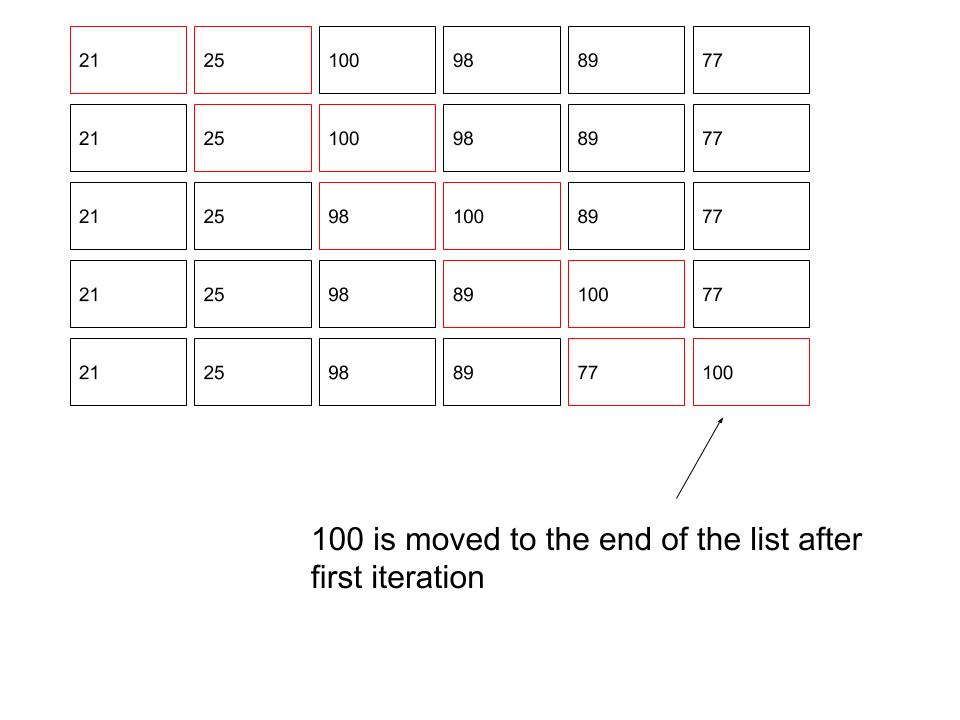# #Data Structure and Algorithm - Bubble Sort

In this tutorial, we will learn a simple sorting algorithm - Bubble Sort.

## #Problem to Solve

Given a list of numbers as shown below, please sort them in ascending order.

``````\$numbers = [21,25,100,98,89,77];
``````

Requirements:

• You are required to use Bubble Sort algorithm when sorting the numbers.
• You are required to implement the algorithm in PHP language.

## #Pseudocode

Bubble Sort works by comparing two values at a time and does it pair by pair. And it iterates until all elements are in place. After each iteration, at least one element is moved to the end of the list. Below is an illustration of the first iteration.Pseudocode of Bubble Sort algorithm can be written as follows:

``````FOR each element of the list

FOR each element of the list

IF current element greater then next element

swap the elements

END IF

END FOR

END FOR
``````

The inner loop is considered one iteration and the outer loop makes sure we iterate enough times to sort the list.

## #PHP Implementation

To implement Bubble Sort in PHP, all we need is two loops. Note that the termination of both loops is: the length of the list - 1. This is to prevent from accessing un-indexed element.

``````<?php
\$numbers = [21, 25, 100, 98, 89, 77];

for (\$i = 0; \$i < (count(\$numbers) - 1); \$i++) {

for (\$j = 0; \$j < (count(\$numbers) - 1); \$j++) {

if (\$numbers[\$j] > \$numbers[\$j + 1]) {

\$tmp = \$numbers[\$j];

\$numbers[\$j] = \$numbers[\$j + 1];

\$numbers[\$j + 1] = \$tmp;
}
}
}

print_r(\$numbers);

// Output:
/*
Array
(
 => 21
 => 25
 => 77
 => 89
 => 98
 => 100
)
*/
``````